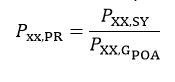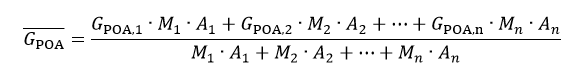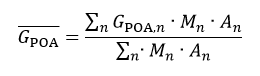# Output

The tool generates an hourly (or sub-hourly) time series of numerous intermediate variables, culminating in the total energy delivered to the grid. In addition, it generates “annual” roll-ups and summary statistics such as specific yield, performance ratio, total generation annual generation, and can supply multi-year summary reports. An account of the annualized “loss factors” are also reported as described below.

# Loss Factor Calculations

Loss factors are computed on a Block by Block basis. A final weighted average is also provided where applicable. The reference points for each of set loss factors quotients are shown in small caps text.### Figure 43. Loss Factor Definitions

The non-STC losses (loss due to lower efficiency at lower irradiance levels, and the loss due to the module temperature coefficient), are detailed as follows:

Reference: Compute the array Pmp at STC conditions (1000 W/m2, 25 °C, air mass 1.5, incidence angle of 90 degrees) and scaled to the effective irradiance. Let this be denoted by Pmp,ηSTC: E.g., @ 200 W/m2, energy output shall be defined as 1/5 the energy at STC. In other words, we assume a linear irradiance dependency of module power.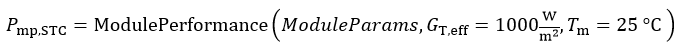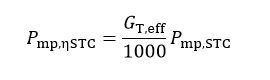Irradiance Loss: Compute the Pmp under actual irradiance conditions but with module temperature fixed at 25 °C. The difference between this Pmp and the previous Pmp will be the energy lost (or gained) due to the module’s response at different radiant intensities.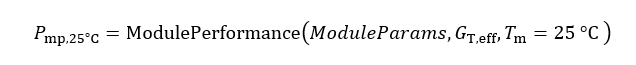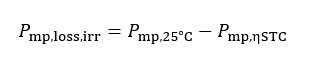# Module Temperature Loss

Temperature loss: Compute the Pmp under real conditions (actual temperature and irradiance). The difference between this Pmp and the previous Pmp will be the energy lost (or gained) due to the modules temperature response; i.e. operation at temperatures other than 25 °C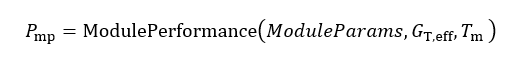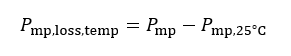# Error Calculation

Given resource and modeling errors input by the user when setting up the simulation parameters, PlantPredict will estimate up to four first year probabilities of exceedance of various annualized metrics. The sources of uncertainty are given in Table 2.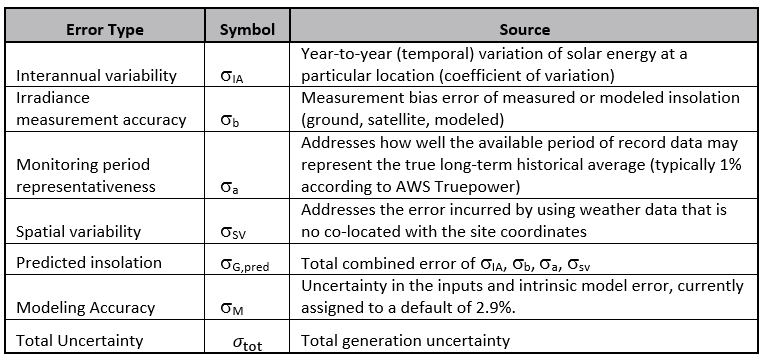### Table 2. Sources of Error

Global horizontal and plane-of-array irradiance uncertainty is computed as follows. For the 1-year values reported in the summary report, the number of years, N, is set to 1: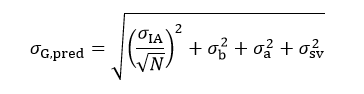The total generated uncertainty affecting DC specific yield, AC capacity factor, Performance Ratio (PR), and total energy, among others, also includes PlantPredict’s internal modeling error: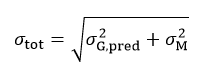The lower confidence interval for all energy metrics, for a desired confidence level (P90, P95) is then computed as follows: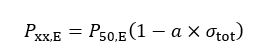Where α is the Z-score for the desired confidence interval and the P50,E is the mean value of the energy metric evaluated. The energy metrics are:

1. Specific Yield
2. AC Capacity Factor
3. Average Array Net Energy
4. Power Plant Net Energy
5. Balance of System (BOS) Losses
6. Non-Netted BOS Station Power

The irradiance metric (GHI and POAI) exceedance probabilities, Pxx,G , are similarly computed, but just applying the irradiance uncertainty to the mean annual irradiance value, P50,G :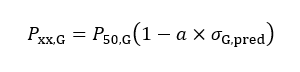The Performance Ratio (PR) is a special case because it is dependent on the plane-of-array irradiance. It is the specific yield at the desired P-level divided by the plane-of-array irradiance at the desired P-level.